Double-slit experiment

﻿
Double-slit experiment

The double-slit experiment, sometimes called Young's experiment, is a demonstration that matter and energy can display characteristics of both waves and particles. In the basic version of the experiment, a coherent light source such as a laser beam illuminates a thin plate pierced by two parallel slits, and the light passing through the slits is observed on a screen behind the plate. The wave nature of light causes the light waves passing through the two slits to interfere, producing bright and dark bands on the screen — a result that would not be expected if light consisted strictly of particles. However, at the screen, the light is always found to be absorbed as though it were composed of discrete particles or photons. This establishes the principle known as wave–particle duality.

Overview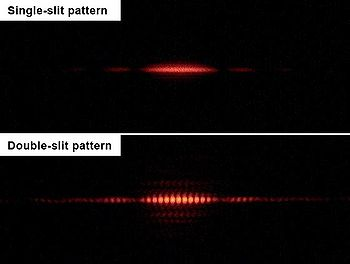Same double-slit assembly (0.7mm between slits); in top image, one slit is closed. Note that the single-slit diffraction pattern — the faint spots on either side of the main band — is also seen in the double-slit image, but at twice the intensity and with the addition of many smaller interference fringes.

If light consisted strictly of ordinary or classical particles, and these particles were fired in a straight line through a slit and allowed to strike a screen on the other side, we would expect to see a pattern corresponding to the size and shape of the slit. However, when this "single-slit experiment" is actually performed, the pattern on the screen is a diffraction pattern, a fairly narrow central band with dimmer bands parallel to it on each side. (See the top photograph to the right.)

Similarly, if light consisted strictly of classical particles and we illuminated two parallel slits, the expected pattern on the screen would simply be the sum of the two single-slit patterns. In actuality, however, the pattern becomes wider and much more detailed, including a series of light and dark bands. (See the bottom photograph to the right.) When Thomas Young first demonstrated this phenomenon, it indicated that light consists of waves, as the distribution of brightness can be explained by the alternately additive and subtractive interference of wavefronts. Young's experiment played a vital part in the acceptance of the wave theory of light in the early 1800s, vanquishing the corpuscular theory of light proposed by Isaac Newton, which had been the accepted model of light propagation in the 17th and 18th centuries. However, the later discovery of the photoelectric effect demonstrated that under different circumstances, light can behave as if it is composed of discrete particles. These seemingly contradictory discoveries made it necessary to go beyond classical physics and take the quantum nature of light into account.

A double-slit experiment was not performed with anything other than light until 1961, when Clauss Jönsson of the University of Tübingen performed it with electrons. In 2002, Jönsson's double-slit experiment was voted "the most beautiful experiment" by readers of Physics World.

In 1999, objects large enough to be seen under a microscope — buckyball molecules (diameter about 0.7 nm, nearly half a million times larger than a proton) — were found to exhibit wave-like interference.

The double-slit experiment (and its variations) has become a classic thought experiment for its clarity in expressing the central puzzles of quantum mechanics. Richard Feynman was fond of saying that all of quantum mechanics can be gleaned from carefully thinking through the implications of this single experiment.

Variations of the experiment

Interference of individual particles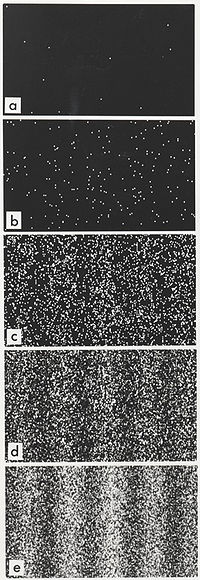Electron buildup over time

An important version of this experiment involves single particles (or waves — for consistency, they are called particles here). Sending particles through a double-slit apparatus one at a time results in single particles appearing on the screen, as expected. Remarkably, however, an interference pattern emerges when these particles are allowed to build up one by one (see the image to the right). For example, when a laboratory apparatus was developed that could reliably fire one electron at a time through the double slit, the emergence of an interference pattern suggested that each electron was interfering with itself, and therefore in some sense the electron had to be going through both slits at once — an idea that contradicts our everyday experience of discrete objects. This phenomenon has also been shown to occur with atoms and even some molecules, including buckyballs. So experiments with electrons add confirmatory evidence to the view of Dirac that electrons, protons, neutrons, and even larger entities that are ordinarily called particles nevertheless have their own wave nature and even their own specific frequencies.

This experimental fact is highly reproducible, and the mathematics of quantum mechanics (see below) allows us to predict the exact probability of an electron striking the screen at any particular point. However, the electrons do not arrive at the screen in any predictable order. In other words, knowing where all the previous electrons appeared on the screen and in what order tells us nothing about where any future electron will hit, even though the probabilities at specific points can be calculated. Thus, we have the appearance of a seemingly causeless selection event in a highly orderly and predictable formulation of the interference pattern. This has prompted some theorists to look for additional determinants or "hidden variables" in the system that, were they to become known, would account for the location of each individual impact with the target.

A low-intensity double-slit experiment was first performed by Taylor in 1909, by reducing the level of incident light until photon emission/absorption events were mostly nonoverlapping. The appearance of interference built up from individual photons could be explained by understanding that a single photon has its own wavefront that passes through both slits, and that the single photon will show up on the detector screen according to the net probability values resulting from the co-incidence of the two probability waves coming by way of the two slits. Note that it is not the probabilities of photons appearing at various points along the detection screen that add or cancel, but the amplitudes. If there is a cancellation of waves at some point, that does not mean that a photon disappears; it only means that the probability of a photon's appearing at that point will decrease, and the probability that it will appear somewhere else increases.

A detailed treatment of the mathematics of double-slit interference in the context of quantum mechanics is given in the article on Englert–Greenberger duality.

With particle detectors at the slits

The double-slit apparatus can be modified by adding particle detectors positioned at the slits. This enables the experimenter to find the position of a particle not when it impacts the screen, but rather, when it passes through the double-slit — did it go through only one of the slits, as a particle would be expected to do, or through both, as a wave would be expected to do? Numerous experiments have shown, however, that any modification of the apparatus that can determine which slit a particle passes through reduces the visibility of interference at the screen, thereby illustrating the complementarity principle: that light (and electrons, etc.) can behave as either particles or waves, but not both at the same time. An experiment performed in 1987 produced results that demonstrated that information could be obtained regarding which path a particle had taken, without destroying the interference altogether. This showed the effect of measurements that disturbed the particles in transit to a lesser degree and thereby influenced the interference pattern only to a comparable extent.

There are many methods to determine whether a photon passed through a slit, for instance by placing an atom at the position of each slit. Interesting experiments of this latter kind have been performed with photons and with neutrons.

Delayed choice and quantum eraser variations

The delayed-choice experiment and the quantum eraser are sophisticated variations of the double-slit with particle detectors placed not at the slits but elsewhere in the apparatus. The first demonstrates that extracting "which path" information after a particle passes through the slits can seem to retroactively alter its previous behavior at the slits. The second demonstrates that wave behavior can be restored by erasing or otherwise making permanently unavailable the "which path" information.

Other variations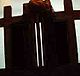A laboratory double-slit assembly; distance between top posts approximately one inch.

In 1967 Pfleegor and Mandel demonstrated two-source interference using two separate lasers as light sources.

It was shown experimentally in 1972 that in a double-slit system where only one slit was open at any time, interference was nonetheless observed provided the path difference was such that the detected photon could have come from either slit. The experimental conditions were such that the photon density in the system was much less than unity.

The experiment has been performed with particles as large as C60 (Buckminsterfullerene).

Classical wave-optics formulationTwo slits diffraction pattern by a plane wave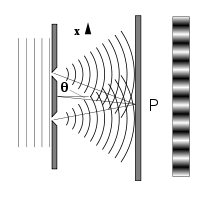Two slits are illuminated by a plane waveGeometry for far field fringes

Much of the behaviour of light can be modelled using classical wave theory. The Huygens–Fresnel principle is one such model; it states that each point on a wavefront generates a secondary spherical wavelet, and that the disturbance at any subsequent point can be found by summing the contributions of the individual wavelets at that point. This summation needs to take into account the phase as well as the amplitude of the individual wavelets. It should be noted that only the intensity of a light field can be measured – this is proportional to the square of the amplitude.

In the double-slit experiment, the two slits are illuminated by a single laser beam. If the width of the slits is small enough (less than the wavelength of the laser light), the slits diffract the light into cylindrical waves. These two cylindrical wavefronts are superimposed, and the amplitude, and therefore the intensity, at any point in the combined wavefronts depends on both the magnitude and the phase of the two wavefronts. The difference in phase between the two waves is determined by the difference in the distance travelled by the two waves.

If the viewing distance is large compared with the separation of the slits (the far field), the phase difference can be found using the geometry shown in the figure below right. The path difference between two waves travelling at an angle θ is given by:$d \sin \theta \approx d \theta$

When the two waves are in phase, i.e. the path difference is equal to an integral number of wavelengths, the summed amplitude, and therefore the summed intensity is maximum, and when they are in anti-phase, i.e. the path difference is equal to half a wavelength, one and a half wavelengths, etc., then the two waves cancel and the summed intensity is zero. This effect is known as interference. The interference fringe maxima occur at angles$~ d \theta_n = n \lambda,~ n=0,1,2,\ldots$

where λ is the wavelength of the light. The angular spacing of the fringes is θf is given by$\theta _f \approx \lambda / d$

The spacing of the fringes at a distance z from the slits is given by$~w=z \theta_f = z \lambda /d$

For example, if two slits are separated by 0.5mm, and are illuminated with a 0.6μm wavelength laser, the spacing of the fringes viewed at a distance of 1m will be 1.2mm.

If the width of the slits b is greater than the wavelength, the Fraunhofer diffraction equation gives the intensity of the diffracted light as:\begin{align} I(\theta) &\propto \cos^2 \left [{\frac {\pi d \sin \theta}{\lambda}}\right]~\mathrm{sinc}^2 \left [ \frac {\pi b sin \theta}{\lambda} \right] \end{align}

This is illustrated in the figure above, where the first pattern is the diffraction pattern of a single slit, given by the sinc function in this equation, and the second figure shows the combined intensity of the light diffracted from the two slits, where the cos function represent the fine structure, and the coarser structure represents diffraction by the individual slits as described by the sinc function.

Similar calculations for the near field can be done using the Fresnel diffraction equation. As the plane of observation gets closer to the plane in which the slits are located, the diffraction patterns associated with each slit decrease in size, so that the area in which interference occurs is reduced, and may vanish altogether when there is no overlap in the two diffracted patterns.

Interpretations of the experiment

Like the Schrödinger's cat thought experiment, the double-slit experiment is often used to highlight the differences and similarities between the various interpretations of quantum mechanics.

Copenhagen interpretation

The Copenhagen interpretation is a consensus among some of the pioneers in the field of quantum mechanics that it is undesirable to posit anything that goes beyond the mathematical formulae and the kinds of physical apparatus and reactions that enable us to gain some knowledge of what goes on at the atomic scale. One of the mathematical constructs that enables experimenters to predict very accurately certain experimental results is sometimes called a probability wave. In its mathematical form it is analogous to the description of a physical wave, but its "crests" and "troughs" indicate levels of probability for the occurrence of certain phenomena (e.g., a spark of light at a certain point on a detector screen) that can be observed in the macro world of ordinary human experience.

The probability "wave" can be said to "pass through space" because the probability values that one can compute from its mathematical representation are dependent on time. One cannot speak of the location of any particle such as a photon between the time it is emitted and the time it is detected simply because in order to say that something is located somewhere at a certain time one has to detect it. The requirement for the eventual appearance of an interference pattern is that particles be emitted, and that there be a screen with at least two distinct paths for the particle to take from the emitter to the detection screen. Experiments observe nothing whatsoever between the time of emission of the particle and its arrival at the detection screen. If a ray tracing is then made as if a light wave (as understood in classical physics) is wide enough to take both paths, then that ray tracing will accurately predict the appearance of maxima and minima on the detector screen when many particles pass through the apparatus and gradually "paint" the expected interference pattern.

Path-integral formulation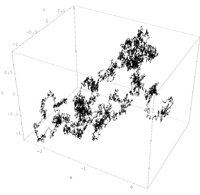One of an infinite number of equally likely paths used in the Feynman path integral. (see also: Wiener process.)

The Copenhagen interpretation is similar to the path integral formulation of quantum mechanics provided by Feynman. The path integral formulation replaces the classical notion of a single, unique trajectory for a system, with a sum over all possible trajectories. The trajectories are added together by using functional integration.

Each path is considered equally likely, and thus contributes the same amount. However, the phase of this contribution at any given point along the path is determined by the action along the path (see Euler's formula):

Apath(x,t) = eiS(x,t)

All these contributions are then added together, and the magnitude of the final result is squared, to get the probability distribution for the position of a particle:$p(x,t) \propto \left\vert \int_{all paths(x,t)} e^{i S(x,t)} \right\vert ^2$

As is always the case when calculating probability, the results must then be normalized:$\int_{x \in X}p(x,t)\,dx = 1$

To summarize, the probability distribution of the outcome is the normalized square of the norm of the superposition, over all paths from the point of origin to the final point, of waves propagating proportionally to the action along each path. The differences in the cumulative action along the different paths (and thus the relative phases of the contributions) produces the interference pattern observed by the double-slit experiment. Feynman stressed that his formulation is merely a mathematical description, not an attempt to describe a real process that we cannot measure.

Relational interpretation

According to the relational interpretation of quantum mechanics, first proposed by Carlo Rovelli, observations such as those in the double-slit experiment result specifically from the interaction between the observer (measuring device) and the object being observed (physically interacted with), not any absolute property possessed by the object. In the case of an electron, if it is initially "observed" at a particular slit, then the observer–particle (photon–electron) interaction includes information about the electron's position. This partially constrains the particle's eventual location at the screen. If it is "observed" (measured with a photon) not at a particular slit but rather at the screen, then there is no "which path" information as part of the interaction, so the electron's "observed" position on the screen is determined strictly by its probability function. This makes the resulting pattern on the screen the same as if each individual electron had passed through both slits. It has also been suggested that space and distance themselves are relational, and that an electron can appear to be in "two places at once" – for example, at both slits – because its spatial relations to particular points on the screen remain identical from both slit locations.

Wikimedia Foundation. 2010.

Look at other dictionaries:

• Photon dynamics in the double-slit experiment — The dynamics of photons in the double slit experiment describes the relationship between classical electromagnetic waves and photons, the quantum counterpart of classical electromagnetic waves, in the context of the double slit experiment. The… …   Wikipedia

• Wheeler's delayed choice experiment — Quantum mechanics Uncertainty principle …   Wikipedia

• Quantum eraser experiment — In physics, the quantum eraser experiment is a double slit experiment that demonstrates several laws of quantum mechanics, including wave particle duality, which seeks to explain certain wave and particle properties of matter, complementarity,… …   Wikipedia

• Afshar experiment — The Afshar experiment is an optical experiment which may challenge the principle of complementarity in quantum mechanics, although there is as yet no consensus on this in physics. The result of the experiment, which was first devised and carried… …   Wikipedia

• N-slit interferometer — The N slit interferometer is an extension of the double slit interferometer also known as Young s double slit interferometer. One of the first known uses of N slit arrays in optics was illustrated by Newton. In the first part of last century,… …   Wikipedia

• Davisson–Germer experiment — Quantum mechanics Uncertainty principle …   Wikipedia

• Delayed choice quantum eraser — Quantum mechanics Uncertainty principle …   Wikipedia

• Introduction to quantum mechanics — This article is an accessible, non technical introduction to the subject. For the main encyclopedia article, see Quantum mechanics. Quantum mechanics …   Wikipedia

• light — light1 lightful, adj. lightfully, adv. /luyt/, n., adj., lighter, lightest, v., lighted or lit, lighting. n. 1. something that makes things visible or affords illumination: All colors depend on light. 2. Physics …   Universalium

• Wave–particle duality — Quantum mechanics Uncertainty principle …   Wikipedia